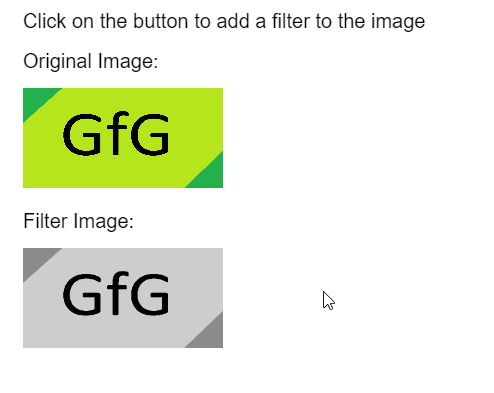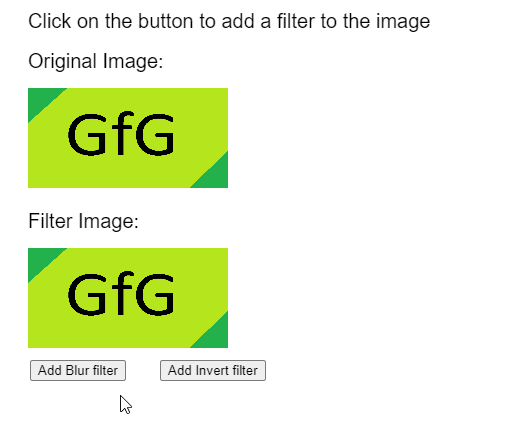# p5.Image filter() Method

• Last Updated : 15 Sep, 2020

The filter() method of p5.Image in JavaScript p5.js library is used to apply the filter to the image. There are several presets predefined in p5.js that can be used with different levels of intensity to get the desired effect.

Syntax:

`filter( filterType, filterParam )`

Parameters: This function accepts two parameters as mentioned above and described below.

• filterType: It is a constant which defines the preset to be used as the filter. It can have the values of THRESHOLD, GRAY, OPAQUE, INVERT, POSTERIZE, BLUR, ERODE, DILATE, or BLUR.
• filterParam: It is a number that is unique to each filter and affects the functionality of the filter. It is an optional parameter.

Note: The JavaScript libraries used in the following examples are as follows. These are used in the head section of any HTML file. The download reference links are given at the bottom of this article.

<script src=”p5.Image.js”></script>
<script src=”p5.min.js”></script>

Example 1: The example below illustrates the filter() method in p5.js.

## javascript

 `function` `preload() { ` `    ``img_orig = ` `      ``loadImage(``"sample-image.png"``); ` `    ``img_filter = ` `      ``loadImage(``"sample-image.png"``); ` `} ` ` `  `function` `setup() { ` `    ``createCanvas(500, 400); ` `    ``textSize(20); ` ` `  `    ``// Draw the original image ` `    ``text(``"Click on the button to "` `+ ` `      ``"add a filter to the image"``, 20, 20); ` `    ``text(``"Original Image:"``, 20, 60); ` `    ``image(img_orig, 20, 80, 200, 100); ` ` `  `    ``// Apply the GRAYSCALE filter ` `    ``img_filter.filter(GRAY); ` ` `  `    ``// Draw the image with filter ` `    ``text(``"Filter Image:"``, 20, 220); ` `    ``image(img_filter, 20, 240, 200, 100);  ` `}`

Output:Example 2:

## javascript

 `function` `preload() { ` `  ``img_orig = ` `    ``loadImage(``"sample-image.png"``); ` `  ``img_filter = ` `    ``loadImage(``"sample-image.png"``); ` `} ` ` `  `function` `setup() { ` `  ``createCanvas(500, 400); ` `  ``textSize(20); ` ` `  `  ``btnBlur = createButton(``"Add Blur filter"``); ` `  ``btnBlur.position(30, 360); ` `  ``btnBlur.mousePressed(applyBlur); ` ` `  `  ``btnInvert = createButton(``"Add Invert filter"``); ` `  ``btnInvert.position(160, 360); ` `  ``btnInvert.mousePressed(applyInvert); ` `} ` ` `  `function` `draw() { ` `  ``clear(); ` ` `  `  ``text(``"Click on the button to add a "` `+ ` `    ``"filter to the image"``, 20, 20); ` `  ``text(``"Original Image:"``, 20, 60); ` `  ``image(img_orig, 20, 80, 200, 100); ` ` `  `  ``text(``"Filter Image:"``, 20, 220); ` `  ``image(img_filter, 20, 240, 200, 100);  ` `} ` ` `  `function` `applyBlur() { ` ` `  `  ``// Add the BLUR filter to the image ` `  ``img_filter.filter(BLUR, 10); ` `} ` ` `  `function` `applyInvert() { ` ` `  `  ``// Add the INVERT filter to the image ` `  ``img_filter.filter(INVERT); ` `}`

Output:Online editor: https://editor.p5js.org/
Environment Setup: https://www.geeksforgeeks.org/p5-js-soundfile-object-installation-and-methods/
Reference: https://p5js.org/reference/#/p5.Image/filter

My Personal Notes arrow_drop_up
Recommended Articles
Page :